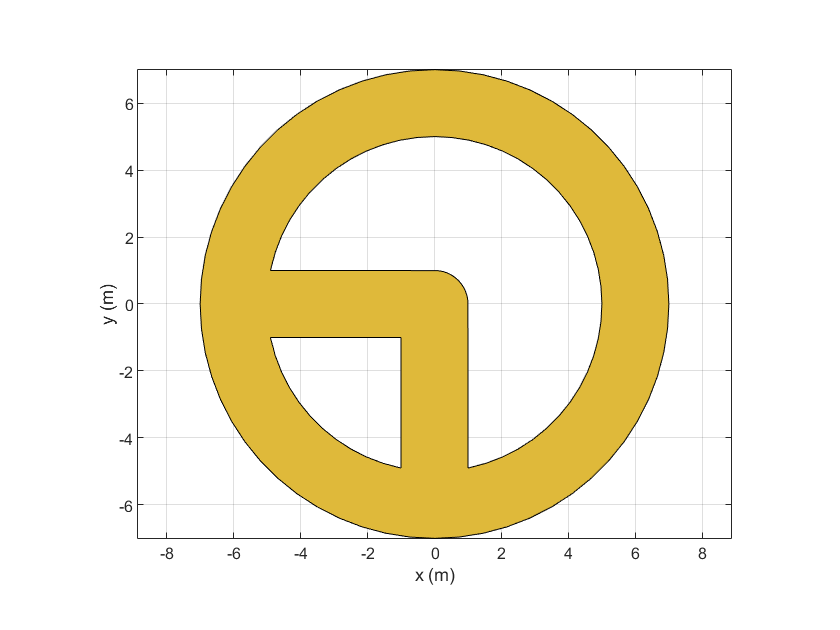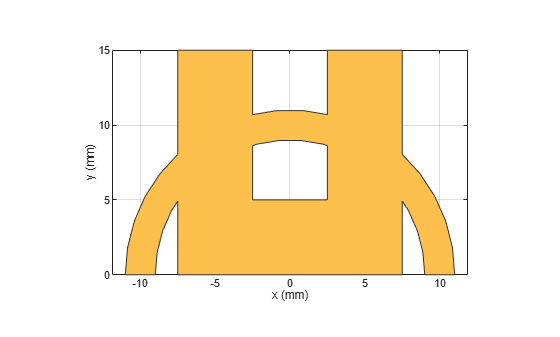# plus

Shape1 + Shape2 for RF PCB shapes

## Syntax

``c = plus(shape1,shape2)``

## Description

example

````c = plus(shape1,shape2)` calls the syntax `shape1 + shape2` to unite two shapes.```

## Examples

collapse all

Create a curved bend shape with a length of 5 m

`bend = bendCurved(Length=[5 5]);`

Create an annular ring shape with the default inner radius of 5 m.

`ring = ringAnnular;`

Add the two shapes and display the result.

`shapeSum = plus(bend,ring)`
```shapeSum = Polygon with properties: Name: 'mypolygon' Vertices: [109x3 double] ```
`show(shapeSum)`Create the default curve shape.

`shape1 = curve;`

Create a right angle U-bend shape with an adjusted size and position to complement the curve shape.

`shape2 = ubendRightAngle;`

Add the two shapes using the + operator, and display the resulting `Polygon` object.

`shapeSum = shape1+shape2`
```shapeSum = Polygon with properties: Name: 'mypolygon' Vertices: [43x3 double] ```
`show(shapeSum)`## Input Arguments

collapse all

First shape created using custom elements and shape objects of RF PCB Toolbox™, specified as an object.

Example: `shape1 = bendCurved;` specifies the first shape as a `bendCurved` object.

Second shape created using custom elements and shape objects of RF PCB Toolbox, specified as an object.

Example: `shape2 = ringAnnular;` specifies the second shape as a `ringAnnular` object.

## Version History

Introduced in R2021b